# Expert Advice on Power Integrity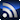RSS

The articles and columns contained in this section come from members of the Signal Integrity Journal’s Editorial Advisory Board (EAB). Members of the EAB review every technical article posted on the site, and are often sought after for their advice. In this column area, the EAB will talk about issues related to power integrity.
Power Integrity

# The Perils of Right-angle Turns at DC

September 1, 2017

Microwave engineers know that sharp corners and right-angle bends have their drawback at high frequencies.  There is a lot of discussion among SI engineers about the impact of right-angle turns in high-speed routing, but it cannot be denied that sharp corners create discontinuities, which eventually could harm the signals by increasing crosstalk, radiation and reflections.

The debate is about the degree of impact, not about the fact.  So what happens if we go to the other extreme of the spectrum, to DC?  Conventional wisdom may suggest that if the problems created by the right-angle discontinuity get worse as frequency goes up, these problems would diminish as the frequency approaches zero.  After all, as we are told, at DC the current penetrates the conductors uniformly.  As it turns out, however, the right-angle turns harm the DC distribution equally badly, if not more, as they harm high-speed signals.  This article shows you why and how, and we will also show simple remedies.

We know that static electricity likes corners and pointed shapes, where like in pockets, it can build up and eventually can cause static discharge (ESD) events.  The biggest ESD events in nature are the lightning strikes and the lightning rods make use of the fact that static charge will accumulate around pointed tips.  But when we consider what happens when all that charge starts to move and creates current, we will notice something interesting.

For instance,  mentions excessive magnetic force and current crowding at right-angle bends in lightning protection metal structures.  All this happens in spite of the fact that the voltage and current profiles of a typical lightning strike are all concentrated at ‘low’ frequencies and the energy quickly tapers off at high frequencies.  True, we can hear the characteristics sound of lightning strikes in atmospheric noise on old-fashioned AM radios at hundreds of kHz frequencies, but that is nowhere near the multi-gigahertz frequencies where we may really be concerned about right-angle bends in our high-speed signals.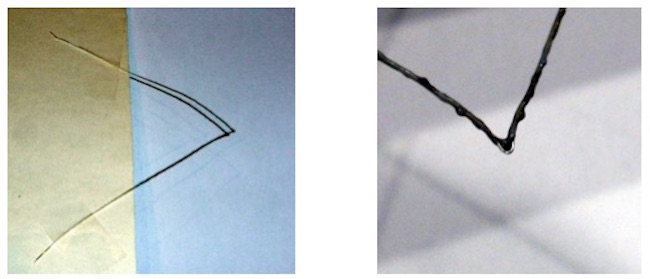Figure 1: 30-gauge solid wire mounted at the edge of a cardboard sheet with (originally) white insulation with a sharp bend in the middle, after exposed to several ampere of DC current.  Left: full view; right: close-up of the bend.

Before we explain what is happening, let’s do a little experimentation that you can also try at home (but be careful not to set your home on fire!).  We can take a uniform conductor, like a straight hookup wire or resistance (heater) wire, make a sharp bend at one point and connect it to a DC current source.  At home you can use D-size batteries and you can play with the number of batteries in series to adjust the current.

For the photos in Figure 1, I used an 8-inch long piece of 30-gauge (0.01 inch or 0.254 mm diameter) wire with white plastic insulation and four D-size batteries. The wire was sharply bent in the middle and was taped to a cardboard sheet to hold it. The four batteries were connected across the ends. After a few seconds, you can see the insulation turning brown and later black, always starting at the bend. If you keep the current flowing, the burn will progress further away from the bend. On the left you see the wire with its burnt insulation. The photo on the right is a close-up at the bend. Note that at the tip the insulation is completely missing, melted away. If you wait long enough, you will also see the wire smoking and eventually melting and opening up at the bend.

Of course this is not a very scientifically controlled experiment. There are many parameters that we may not know, like how much cooling occurs through the attachments.  Some other parameters we may not be able to control very well. For instance, in the simple home-made experiment, the current was adjusted experimentally by changing the number of stacked batteries, but I did not measure their internal resistance, which in turn could be different for each piece. However, on an average, even with this simple experiment, we will see signs of the highest temperature occurring at the bend. In a lab, for more convincing results, you can use thicker wires with higher current or right-angle surface PCB traces with adjustable bench supplies, where you can conveniently set the current.

To understand what is happening, we need to start at the basics. For current flow and thermal effects in PCBs, a good summary to read is .  Figure 2 shows a typical scenario we may use when it comes to the resistance and DC current flow in traces.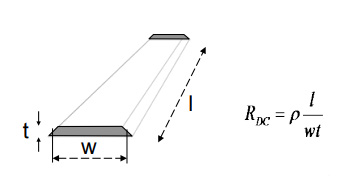Figure 2: Cross section and resistance calculation of a PCB trace.

If we need to calculate the DC resistance of a straight trace with uniform cross section along its length between the grey surfaces, the formula tells us to multiply the resistivity of the material with the trace length and divide by the cross sectional area.

There is, however, a hidden assumption or condition that comes with this formula, something that we may easily forget about. The formula gives the correct result only if the current density is uniform through the cross section surfaces where the current enters and exits the trace.  When we calculate the resistance of a trace, we usually don’t need to worry about this condition, because we typically want to calculate the resistance for cases when the length is much bigger than the cross sectional dimensions. Even if the current density is not uniform at the entry and exit surfaces, the current will redistribute itself within a distance equaling a few times the trace width (or height, whichever is bigger), so the portion of the total length with non-uniform current density will be negligibly small.

When the length-to-width aspect ratio is not very big, like on plane shapes, or when the cross section changes, for instance we have a turn, we need more detailed calculations.  The formula in Figure 2 still holds for short aspect ratio pieces, like plane shapes, if the uniformity of current density is maintained through the entry and exit surfaces.  Figure 3 shows a small aspect ratio rectangular plane shape and its DC resistance formula, assuming that current enters and exits on the grey surfaces on the left and right.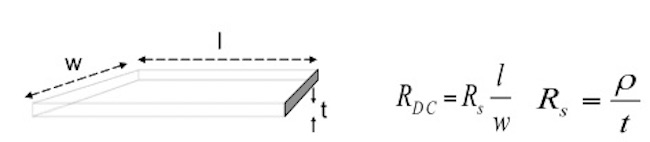Figure 3: Cross section and resistance of a rectangular plane shape.

If we have to calculate the effect of non-uniform current density at the entry and exit surfaces, we can use any of the commercial tools that can calculate DC drop by meshing the metal, see for instance , ,  or . The commercial tools are great to analyze complex geometries with many details and large structures. To study only simple geometries, and to improve our intuition, we can use free tools, like , and a little work.  On the left of Figure 4 we see the topology view of a resistor grid, which can be used to analyze the DC voltage and current on a square plane shape. The plane is discretized, in this case for twenty cells along both the X and Y direction, creating 21 nodes in each way.  The total number of nodes is 21 x 21 = 441.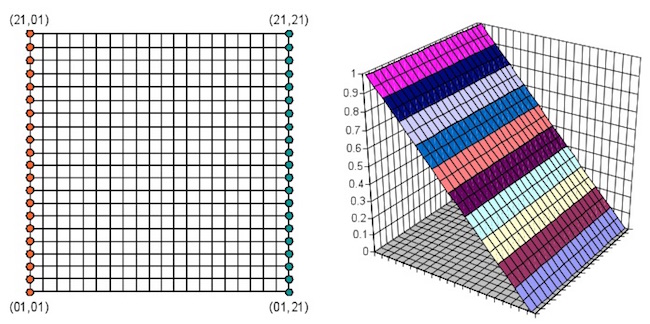Figure 4: Berkeley SPICE model to simulate the current flow in square plane shapes (left) and potential surface (on the right).

Each line represents the resistance of a little piece (1/400th of the area) of the plane and in the SPICE deck it can be represented by a resistance of R. For now, the actual value of R does not really matter, we just have to make sure that each segment has the same resistance value inside the grid, and twice the resistance along the perimeter, which accounts for the fact that on one side of the boundary there is no continuation of the plane.  We have to add voltage or current sources, sinks, and we also have to designate node(s) to SPICE Node 0, to establish a potential reference.

In Figure 4, the source and sink nodes are marked by orange and green dots, respectively. The source uniformly excites the left edge of the plane with a total of 1A DC current. For sake of simplicity, all sink nodes are tied to Node 0. We add SPICE directives to specify the calculations, in this case just the DC operating point, and we are ready to go. This SPICE deck is available for download . (In a later posting I will also give you the solutions how to run Berkeley SPICE on this input circuit.) The DC operating points in the output file created by SPICE are plotted on the right of Figure 4.  The values are normalized to the maximum reading, and we see that the values linearly get smaller as we move on the plane from left to right. This situation corresponds to the case when we maintain constant current density through the entry and exit surfaces, and, therefore, we can apply the simple formulas to calculate the total resistance. Here we don’t plot the current density, simply because it is constant all over the plane.

Now that we established our reference, we can change the source and sink nodes to see what happens when the current density is not uniform at the entry and/or exit areas. Here I want to show you one of my favorite plots from the large collection of cases we go through in my power integrity courses. Figure 5 shows the footprint and graphical representation of the SPICE deck.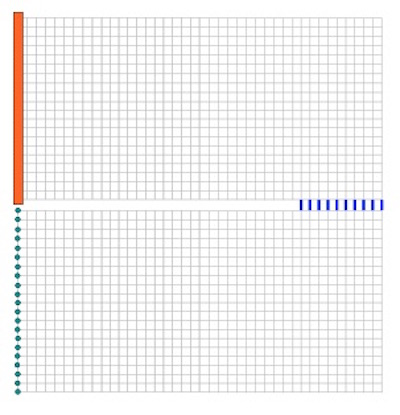Figure 5: SPICE deck graphical representation of a square plane with a slot.

To get a better resolution, here the SPICE deck is expanded to 40 x 40 cells. The plane with a slot is put together from two rectangular planes with 20 x 40 cells. The two rectangular planes are attached and connected together through ten nodes. The ten short blue lines between the top and bottom rectangles represent these ideal electrical connections. As in Figure 4, the orange and green dots represent source and sink connections. At each of the orange dots, we force the same amount of DC current; this ensures that the current density at the entry surface is uniform. For the sake of simplicity, all green sink nodes are connected to Node 0.

Though this will not enforce uniform current density through the exit surface by design, as we see in Figure 6, this geometry eventually will produce quite uniform current density at the sink nodes. In Figure 6, we plot the potential (on the left) and the current (on the right) along the surface of this slotted plane.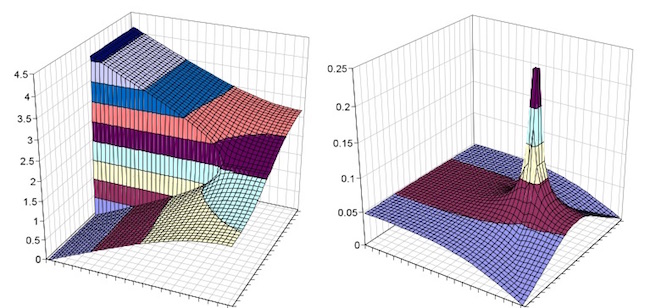Figure 6: Potential surface (on the current) and relative current density (on the right) of the circuit shown in Figure 5.

The potential surface slopes down as expected, almost linearly within each rectangular shape. The first telling sign is that the slope of the potential surface becomes quite steep on the inner corner of the slot.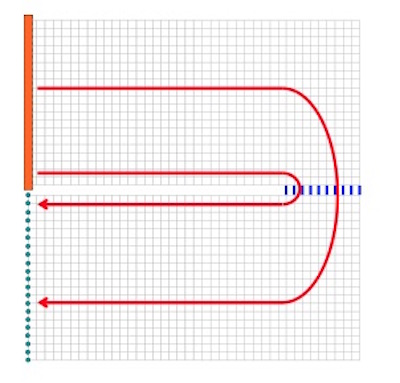Figure 7: SPICE circuit representation with the shortest and with a longer current path marked by red lines.

When we look at the relative current density on the right, we see a huge spike at the same spot. In this rather extreme case it is easy to see why this happens. When the current travels between the source and sink nodes, we can identify a ‘short’ path, which goes right around the inner corner of the slot. All other paths will be longer, hence will have higher resistance (see Figure 7).

At DC, the actual path of the current flow is dictated by the path resistance, so no wonder more current would like to go near the inner corner, where the resistance of the full path is less. This, on the other hand, creates current crowding at the corner, increased IR drop, increased equivalent resistance and increased self-heating.

Interestingly, this looks very similar to the high-frequency behavior of turns and corners in traces.  As Figure 8 of  illustrates, when high-speed signals encounter a change, they seek the shortest path. And as we have just shown, this is exactly what is happening at DC, too.

In case you wonder how to cope with geometry constraints when we need to change the direction of a power strip or need to widen or narrow power planes, a couple of simple suggestions are shown in Figure 8.  For stitching plane shapes with a via array, you can also consult .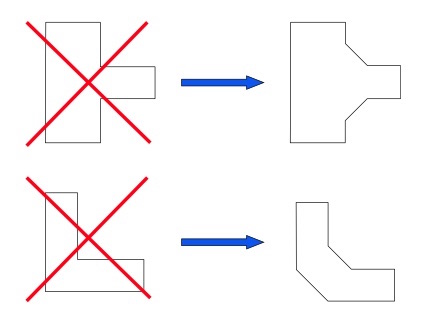Figure 8: Suggested geometries to avoid current crowding at sharp corners.

So when you design your next printed circuit board or a package that may carry large currents, keep in mind that as opposed to static electricity, which likes corners to build up, DC current will stay away from sharp corners.  And if we give the current no choice but to flow around a sharp corner, the current density can be very high at the sudden turns, eventually overstressing and possibly burning the conductor.

References

   “Limits of Lightning Protection Systems,” IEEE EMC Magazine, Vol.5, pp 59-64, Q1 2016

   Douglas Brooks, Johannes Adam: PCB Trace and Via Currents and Temperatures: The Complete Analysis.  2nd Edition. CreateSpace Independent Publishing Platform.  February 2017.